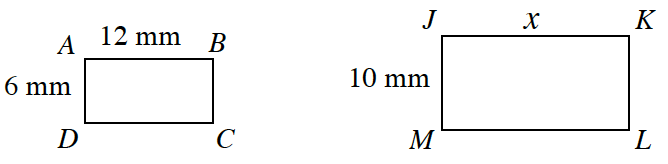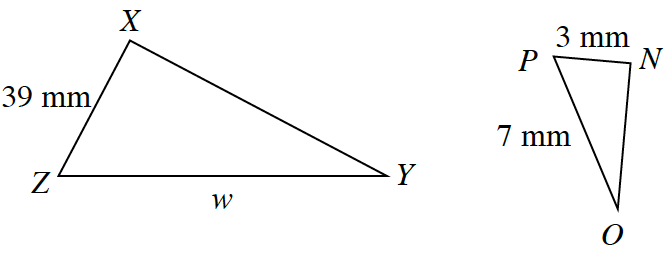### Home > INT2 > Chapter 2 > Lesson 2.3.4 > Problem2-117

2-117.

Solve for the missing lengths in the sets of similar figures below.

1. $ABCD \sim JKLM$If each pair of shapes is similar, then

$\frac{6}{12} = \frac{10}{x} \text{ and }\frac{39}{w} = \frac{3}{7}.$

Start to solve for each variable by multiplying both sides of the equation by the lowest common denominator.

1. $∆NOP \sim ∆XYZ$$w = 91$